Question

# Determine (a) the moment of inertia of .the shaded area about the x axis 2 in....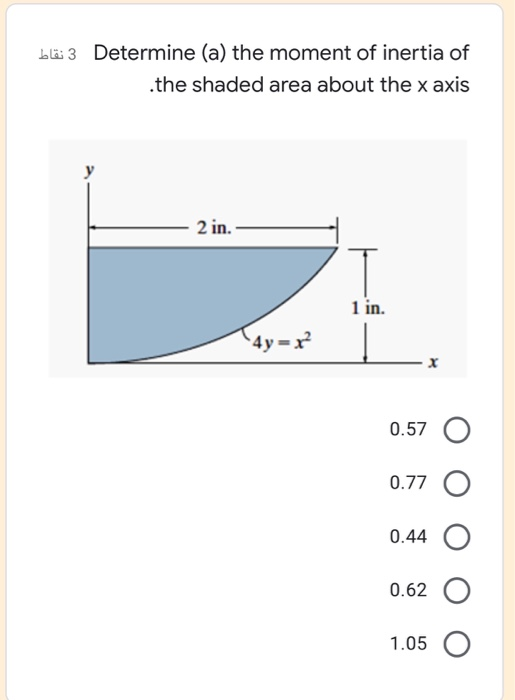Determine (a) the moment of inertia of .the shaded area about the x axis 2 in. 1 in. 4y= = x 0.57 O 0.77 O 0.44 O 0.62 O 1.05 O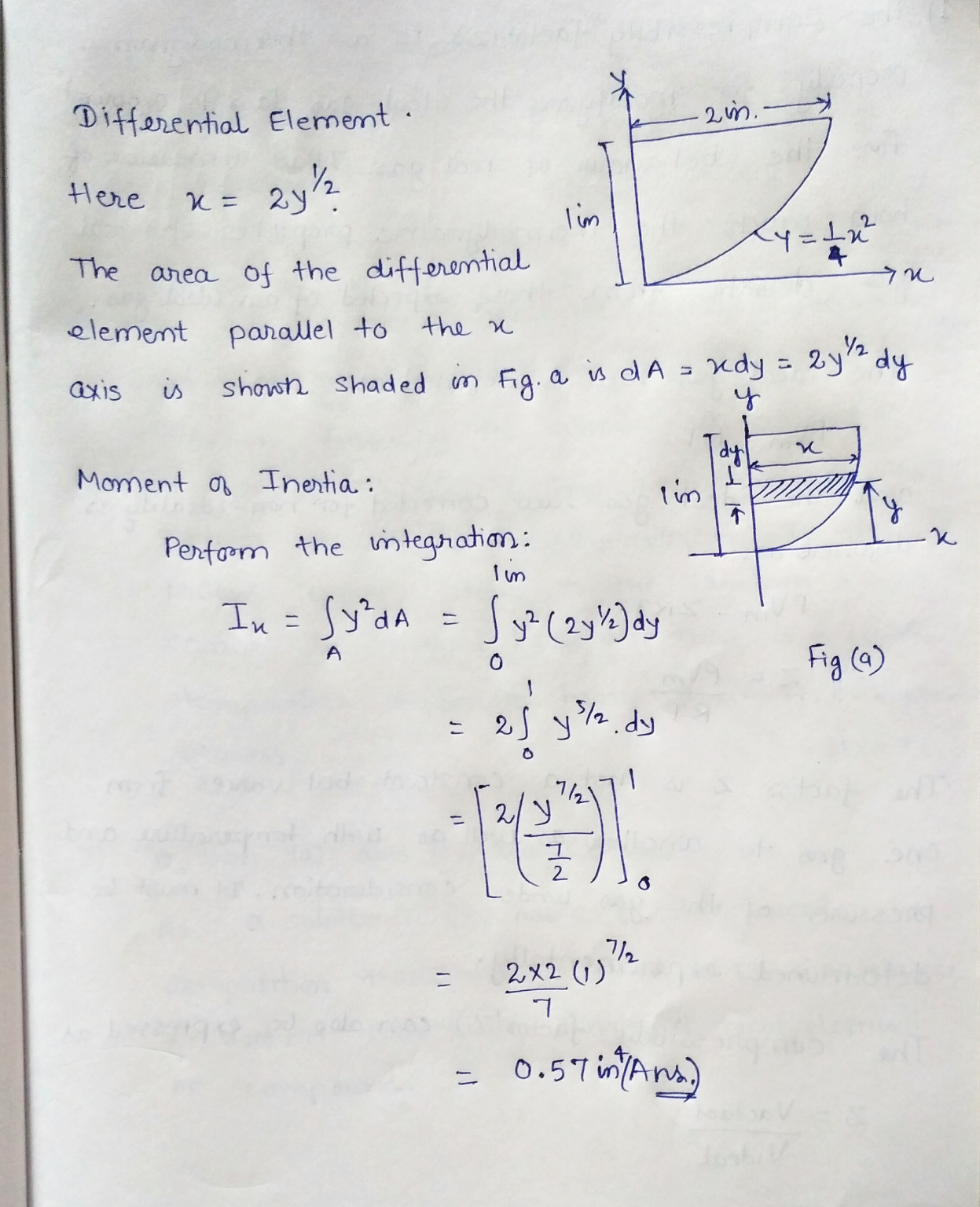#### Earn Coins

Coins can be redeemed for fabulous gifts.

Similar Homework Help Questions
• ### determine the moment of inertia I_ of the shaded area about X axis Determine the moment...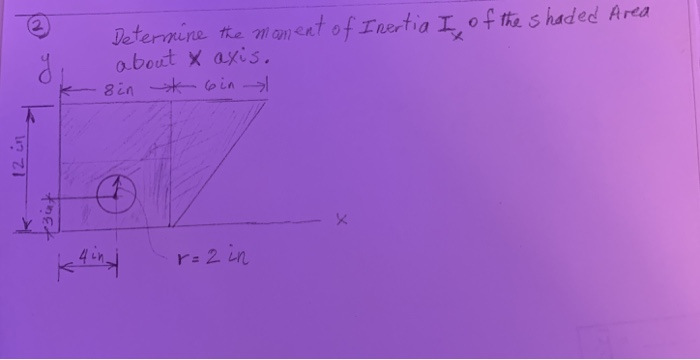determine the moment of inertia I_ of the shaded area about X axis Determine the moment of Inertia I of the shaded Area about X axis. sin t ein kuin r= 2in

• ### Determine the moment of inertia for the shaded area about the x axis.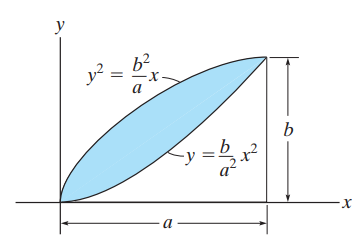Determine the moment of inertia for the shaded area about the x axis.

• ### 3 نقاط b) The moment of inertia of the) shaded area about the y axis 2...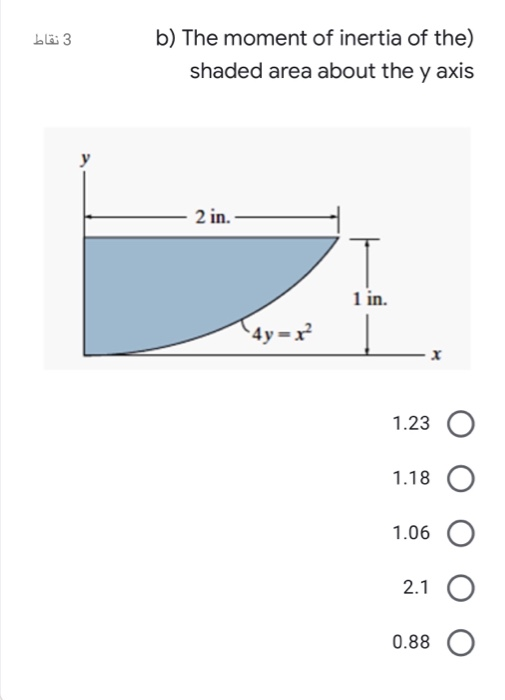3 نقاط b) The moment of inertia of the) shaded area about the y axis 2 in. 1 in. (4y=x 1.23 O 1.18 O 1.06 O 2.1 0.88

• ### Determine the moment of inertia for the shaded area about the x axis. (Figure 1)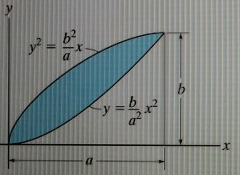Determine the moment of inertia for the shaded area about the x axis. (Figure 1)

• ### 3. Determine the moment of inertia for the shaded area about the x and y axis...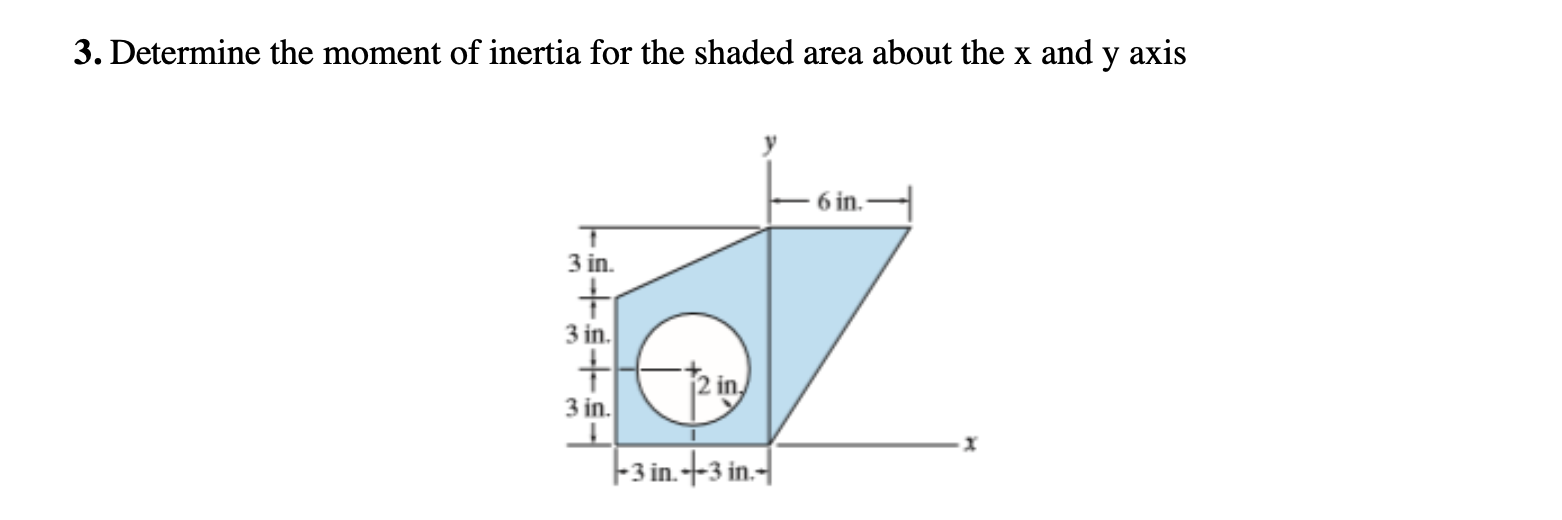3. Determine the moment of inertia for the shaded area about the x and y axis 6 in. 3 in. + 3 in. + 3 in. 1 1-3 in. + 3 in 1

• ### Determine the moment of inertia for the shaded area about the x axis. State the method...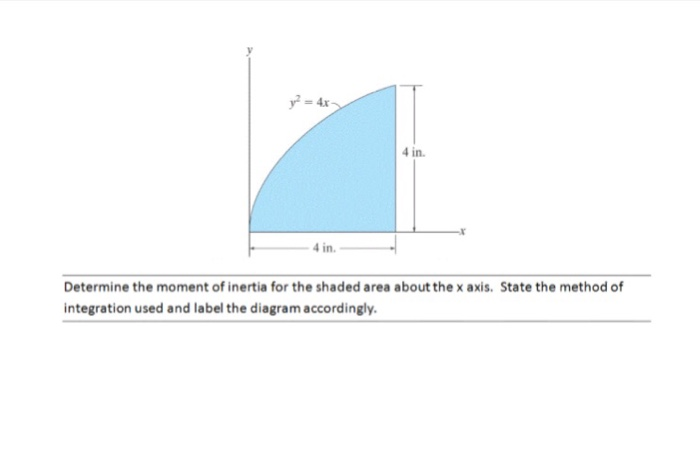Determine the moment of inertia for the shaded area about the x axis. State the method of integration used and label the diagram accordingly.

• ### Determine the moment of inertia of the shaded area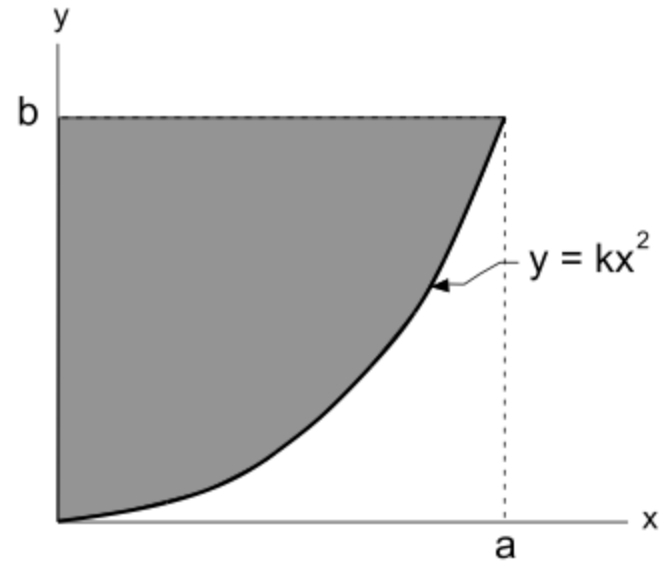Determine the moment of inertia (2nd moment of area) of the shaded area a) about the x-axis, b) about the y axis, and c) about the x’ and y’ axes through the centroid of the shaded area. d) Find the polar moment of inertia Jc about the z’ axis passing through the centroid.

• ### Determine the moment of inertia of the shaded area about the y axis.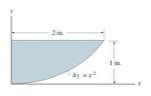Determine the moment of inertia of the shaded area about the y axis.

• ### Determine the moment of inertia for the shaded area about the y axis for the shape...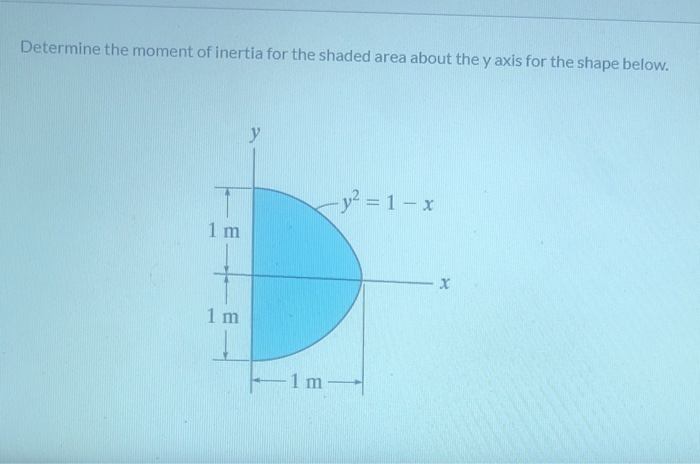Determine the moment of inertia for the shaded area about the y axis for the shape below. у - y² = 1- x 1 m 1 m -1 m

• ### Determine the moment of inertia of the shaded area about the x axis and the y...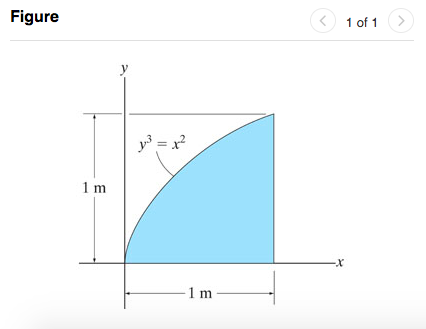Determine the moment of inertia of the shaded area about the x axis and the y axis. Figure < 1 of 1 > 1 m